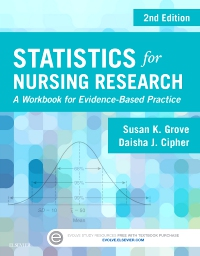ISBN: 9780323376570
Page Count: 480
Imprint: Saunders
List Price: \$50.95## Statistics for Nursing Research – Elsevier eBook on VitalSource, 2nd Edition

by Susan K. Grove, PhD, RN, ANP-BC, GNP-BC and Daisha J. Cipher, PhD
Elsevier eBook on VitalSourceISBN: 9780323376570
Page Count: 480
Imprint: Saunders
List Price: \$50.95This is a Faculty Product!
Any student who attempts to request a review copy will be reported to the school's faculty and administration.
All review copy requests, upon verification of instructor status, will be fulfilled as a digital version of the product, if available.

Help your students understand the statistical methods used in research articles! Statistics for Nursing Research: A Workbook for Evidence-Based Practice, 2nd Edition helps students learn to interpret and analyze the statistical data found in health sciences research articles. Practical exercises show how to critically appraise sampling and measurement techniques, and how to evaluate results. Ideal for undergraduate students, graduate students, and practicing nurses, this is the only statistics workbook for nursing to include research examples from both nursing and medical literature, for a complete perspective on health sciences research.

• Comprehensive coverage includes exercises that address all common techniques of sampling, measurement, and statistical analysis that you are likely to see in nursing and medical literature.
• A literature-based approach incorporates a relevant research article into each exercise/chapter, with key excerpts.
• 45 sampling, measurement, and statistical analysis exercises provide a practical review of both basic and advanced techniques, and prepare you to apply statistics to nursing practice.
• Consistent format for all chapters facilitates quick review and easier learning, covering the statistical technique in review, results from a research article, and study questions.
• Study questions in each chapter help you apply concepts to clinical practice.
• Questions to Be Graded in each chapter may be completed and submitted online, to assess your mastery of key statistical techniques.
• A concise index makes it easy to locate information quickly.
• NEW examples show the latest, high-quality research studies.
• NEW! Expanded coverage helps undergraduate students apply the information learned in statistics and research courses, serves as a refresher/review for graduate students, and also helps in critically appraising studies to determine whether their findings may be used in evidence-based practice.
• NEW! Understanding Statistical Methods section includes exercises to help in understanding the levels of measurement (nominal, ordinal, interval, and ratio) and in appraising the samples and measurement methods in studies.
• NEW! Conducting and Interpreting Statistical Analyses section includes exercises to help in understanding the power analysis and how to conduct a power analysis for a study, showing how to determine the most appropriate statistical method(s) for analyzing data for a class project, for a clinical agency project, or for an actual research study.
• NEW! Answers to study questions are located in the back of the book.
• Part 1: Understanding Statistical Methods
1. Identifying Levels of Measurement: Nominal, Ordinal, Interval, and Ratio
2. Identifying Probability and Nonprobability Sampling Methods in Studies
3. Understanding the Sampling Section of a Research Report: Population, Sampling Criteria, Sample Size, Refusal Rate, and Attrition Rate
4. Understanding Reliability of Measurement Methods
5. Understanding Validity of Measurement Methods
6. Understanding Frequencies and Percentages
7. Interpreting Line Graphs
8. Measures of Central Tendency: Mean, Median, and Mode
9. Measures of Dispersion: Range and Standard Deviation
10. Description of a Study Sample
11. Interpreting Scatterplots
12. Algorithm for Determining the Appropriateness of Inferential Statistical Techniques
13. Understanding Pearson’s Product-Moment Correlation Coefficient
14. Understanding Simple Linear Regression
15. Understanding Multiple Linear Regression
16. Understanding Independent Samples t-test
17. Understanding Paired or Dependent Samples t-test
18. Understanding Analysis of Variance (ANOVA) and Post Hoc Analyses
19. Understanding Chi Square
20. Understanding Spearman Rank-Order Correlation Coefficient
21. Understanding Mann-Whitney U Test
22. Understanding Wilcoxon Matched-Pairs Signed-Ranks Test
Part 2: Conducting and Interpreting Statistical Analyses
23. Selecting Appropriate Analysis Techniques for Studies
24. Describing the Elements of Power Analysis: Standard Power, Effect Size, Alpha, and Sample Size
25. Conducting Power Analysis
26. Determining the Normality of a Distribution
27. Calculating Descriptive Statistics
28. Calculating Pearson's Product-Moment Correlation Coefficient
29. Calculating Simple Linear Regression
30. Calculating Multiple Linear Regression
31. Calculating t-tests for Independent Samples
32. Calculating t-tests for Paired (Dependent) Samples
33. Calculating Analysis of Variance (ANOVA) and Post Hoc Analyses Following ANOVA
34. Calculating Sensitivity and Specificity
35. Calculating Pearson Chi-Square
36. Calculating Odds Ratio and 95% Confidence Intervals
References
Appendix A: Critical Values for Student's t Distribution
Appendix B: Critical Values of r for Pearson Product Moment Correlation Coefficient
Appendix C: Critical Values of F for α = 0.05 and α = 0.01
Appendix D: Critical Values of the χ2 Distribution
• Susan K. Grove, PhD, RN, ANP-BC, GNP-BC, Professor, College of Nursing, The University of Texas at Arlington, Arlington, Texas; Adult Nurse Practitioner, Family Practice, Grand Prairie, Texas and Daisha J. Cipher, PhD, Associate Professor, College of Nursing and Health Innovation, The University of Texas at Arlington, Arlington, Texas
This is a Faculty Product!
Any student who attempts to request a review copy will be reported to the school's faculty and administration.